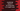# 4 ways in JavaScript to check if the first character of a string is in upper case## JavaScript program to check if the first character of a string is in upper case or not:

In this post, we will learn how to check if the first character of a string is in upper case or not in JavaScript. We will learn different ways to check it in this post.

JavaScript provides built-in methods to help us work with strings. With this post, you will also learn how to capitalize a character in JavaScript.

### Method 1: By comparing the first character with its upper case:

JavaScript doesn’t provide any method to check if a character is in upper case or not. But, it provides a method toUpperCase() to convert a character to upper case. We can pick the first character of a string and compare it with its upper case to check if the character is in upper case or lower case.

There is another method called charAt to get a character of a string. This method takes the index value and returns the character at that index for a string.

So, we can use charAt(0) and toUpperCase() methods to convert the first character of a string to upper case and compare it with the original one.

Let’s write down the complete program:

``````const isStartingUppercase = str => str.charAt(0) === str.charAt(0).toUpperCase();

let arr = ['hello', '123Hello', 'Hello World', '', ' Hello', 'H', 'a', '#@\$'];

arr.forEach(e => console.log(`\${e} => \${isStartingUppercase(e)}`));``````

The array arr contains different types of strings. isStartingUppercase arrow function takes a string as the parameter andd returns a boolean value. It returns true if the first character of str is in upper case and returns false if it is not.

If you run the above program, it will print the below result:

``````hello => false
123Hello => true
Hello World => true
=> true
Hello => true
H => true
a => false
#@\$ => true``````

As you can see, it failed if the string is starting with number, empty character or any other special character. It works only for lowercase and uppercase alphabet.

### Method 2: Capitalize the first character and append it with the rest:

This is another way to check if the first character is upper case or not. We can *capitalize the first character of a string and append it with the rest of the string. If the result is equal to the original string, the first character of that string is in upper case.

``````const isStartingUppercase = str => str === str.charAt(0).toUpperCase() + str.slice(1);

let arr = ['hello', '123Hello', 'Hello World', '', ' Hello', 'H', 'a', '#@\$'];

arr.forEach(e => console.log(`\${e} => \${isStartingUppercase(e)}`));``````

This will give the same output as the previous example.

``````hello => false
123Hello => true
Hello World => true
=> true
Hello => true
H => true
a => false
#@\$ => true``````

This returns false if the first character is a number, empty character, special character. It works only if the first character is an alphabet.

### Method 3: By using charCodeAt:

It is an inbuilt method defined for strings in JavaScript. The charCodeAt method takes the index value as its parameter and returns the character code or UTF-16 code unit for the character at that index position.

We can compare the return value of charCodeAt with 65 and 90. 65 is for ‘A’ and 90 is for ‘Z’. So, if the return value is in this range, the character at that position is in upper case.

``````const isStartingUppercase = str => str.charCodeAt(0) >= 65 && str.charCodeAt(0) <= 90;

let arr = ['hello', '123Hello', 'Hello World', '', ' Hello', 'H', 'a', '#@\$'];

arr.forEach(e => console.log(`\${e} => \${isStartingUppercase(e)}`));``````

It will return the correct results for the test strings.

``````hello => false
123Hello => false
Hello World => true
=> false
Hello => false
H => true
a => false
#@\$ => false``````

It returns true only if the first character of a string is in upper case. Otherwise, it returns false.

### Method 4: By using regular expression, regex:

A regular expression can be used to check if the first character of a string is in upper case or not. We can use the regular expression /[A-Z]/. This should be checked with the first character of the string.

We can use the regular expression with the test() method to check if the first character of a string is in upper case or not.

``````const isStartingUppercase = str => /[A-Z]/.test(str.charAt(0));

let arr = ['hello', '123Hello', 'Hello World', '', ' Hello', 'H', 'a', '#@\$'];

arr.forEach(e => console.log(`\${e} => \${isStartingUppercase(e)}`));``````

It will print:

``````hello => false
123Hello => false
Hello World => true
=> false
Hello => false
H => true
a => false
#@\$ => false``````